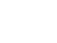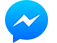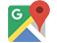﻿How to Calculate Pool Pump Run Time | Dofi Water - Thiết hồ bơi & Spa

22 Nguyen Binh Khiem, Ward 1, Go Vap District, Ho Chi Minh City.
Giỏ Hàng
0969 689 079
Khách hàng

Dofi cung cấp và lắp đặt thiết bị hồ bơi, thiết bị xử lý nước, công nghệ làm nóng nước heat & heater pump, hệ thống tưới tự động

tài liệu

#### CÁC VẤN ĐỀ NƯỚC HỒ BƠI THƯỜNG GẶP

video
HỖ TRỢ KHÁCH HÀNG

### Tư vấn kỹ thuật

How to Calculate Pool Pump Run Time

How to Calculate Pool Pump Run Time

If you have a pool, then you have a pool pump. But how long should you run the pump? Knowing that a pool pump filter system is the second highest energy consumer during the summer, (after AC units), we want you to get the most bang for your buck.

Let’s walk through a few common questions and then breakdown the calculation behind finding the ideal pool pump run time for your swimming pool.

What does the pool pump do?
To understand how much run time you need, it’s important to know exactly what your pump does for your pool. The pool pump is the heart of your pool filtration system. It circulates water out and back in your pool, distributing pool chemicals and filtering your water. It is recommended that you “turnover” or circulate all of your pool water at least once throughout the day. This will ensure that the chemicals are evenly distributed and your water stays clean and clear. If you don’t circulate the water, you run the risk of algae growth and unbalanced water. This may lead to water that is unsafe for swimmers, etch, and cause pitting within your pool surfaces.

How many gallons of water are in my pool?
Before we start, let’s understand how many gallons of water are in your pool. To find your pool’s volume, use one of these easy calculations. If you don’t want to calculate the pool volume, take a quick look at the table on this helpful article: Calculating Pool Volume.

Square and Rectangle Pools:

Length x Width x Depth x 7.5 = Volume (Gallons)

Square and Rectangle Pools with variable depths:

Length x Width x Average Depth x 7.5 = Volume (Gallons)

Round Pools:

3.14 x Radius Squared x Average Depth x 7.5 = Volume (Gallons)

Irregular Shaped Pools:

0.45 x (A+B) x Length x Average Depth x 7.5 = Volume (Gallons)

What is the turnover rate?
Now that we have the pool volume in gallons, we can calculate the turnover rate. Most pool pumps go by gallons per minute (GPM), versus gallons per hour (GPH). Here is how to get that number:

Total Pool Volume ÷ 8 = GPH

GPH ÷ 60 = GPM

Let’s do some math!
Now that we have gallons per minute (GPM) and pool volume, we can calculate the run time. The math is simple. For this example, let’s say you have a 20,000 gallon pool and the pool pump has a GPM rating of 40. Grab that calculator and just “sub-in” your measurements for these:

40 (GPM) X 60 (minutes per hour) = 2400 gallons per hour

20,000 (gallons) / 2400 (gallons per hour) = 8.3 hours

In this case, the ideal pool pump run time is 8.3 hours per day for one circulation or “turnover” of water.
BY BRIAN CHAMP –Tin liên quan
Bình luận

### Liên hệSubscribe
Thông báo choGọi điệnSMSLiên hệ